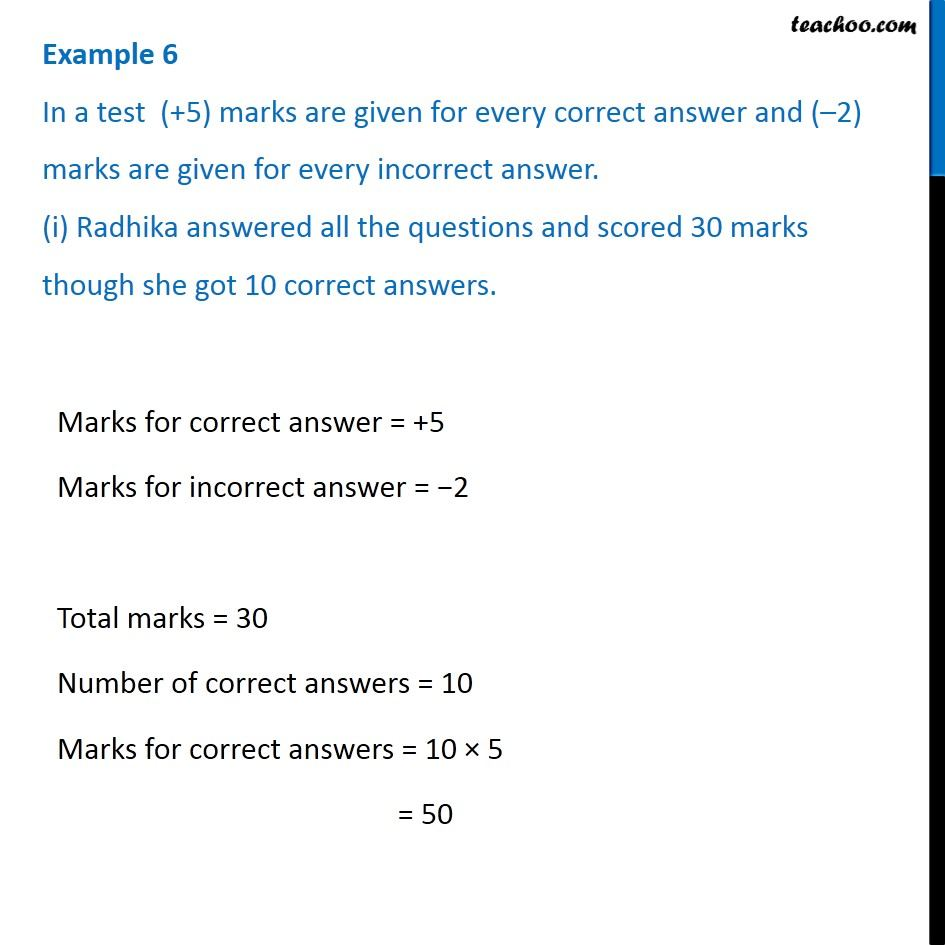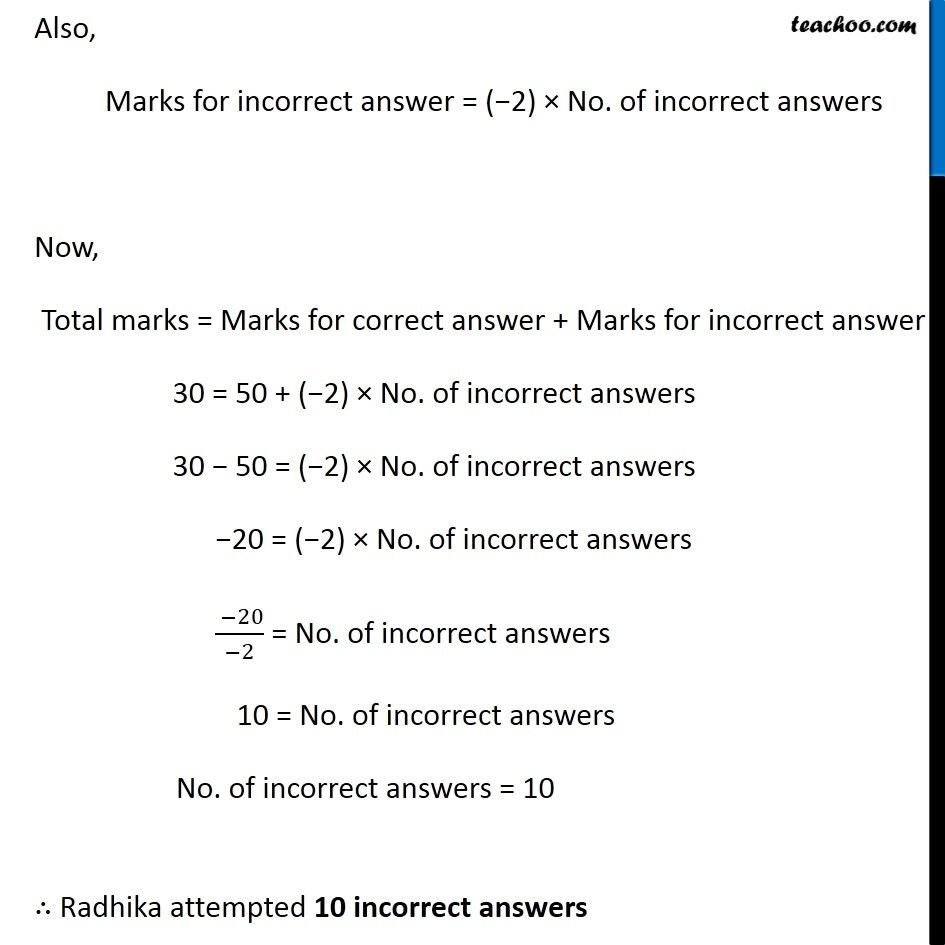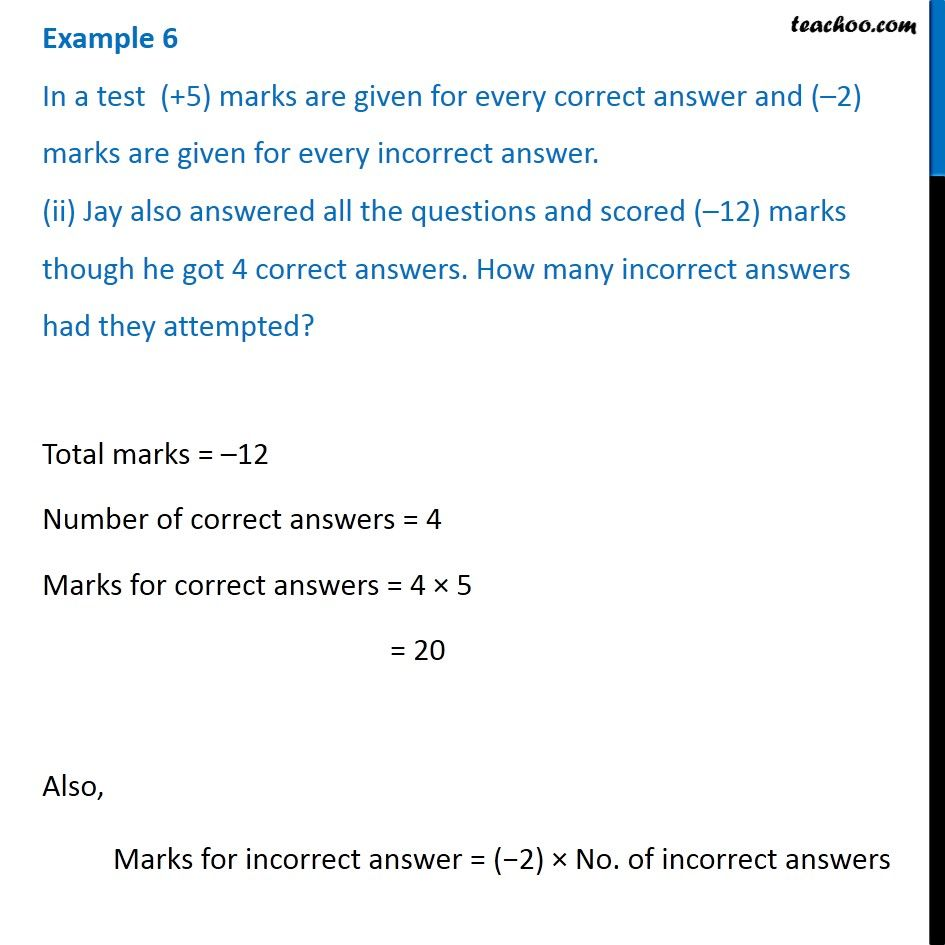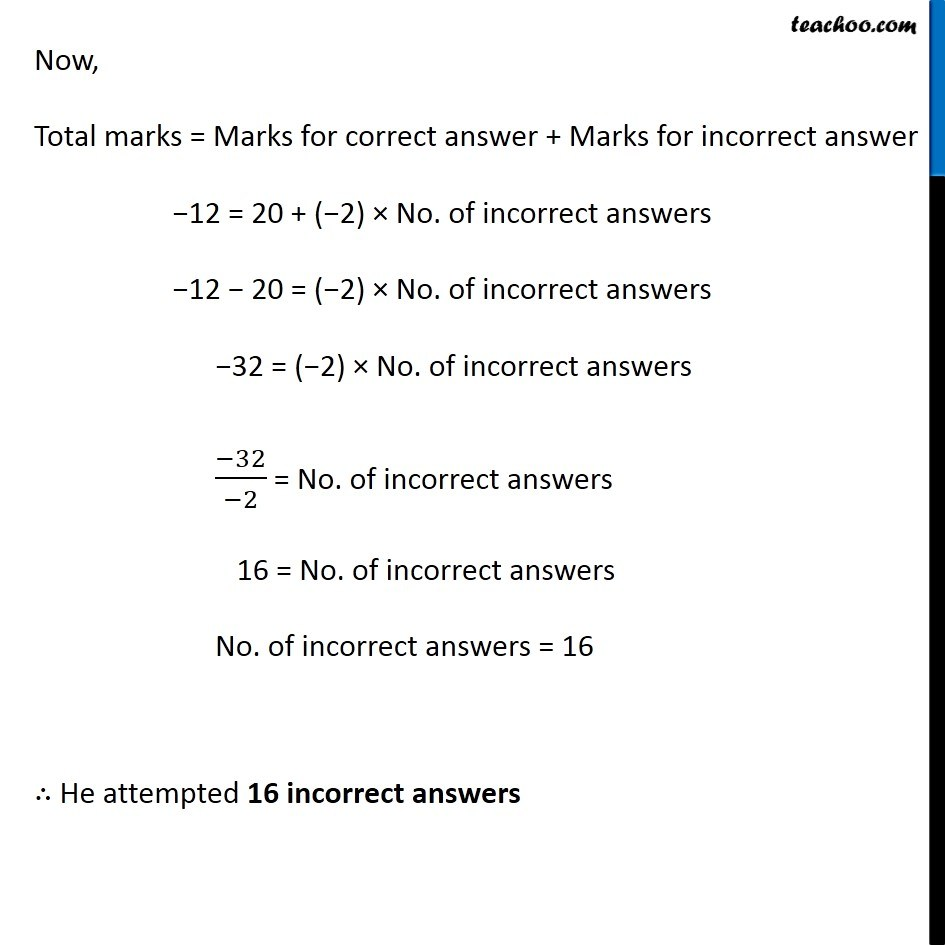1. Chapter 1 Class 7 Integers
2. Serial order wise
3. Examples

Transcript

Example 6 In a test (+5) marks are given for every correct answer and (–2) marks are given for every incorrect answer. (i) Radhika answered all the questions and scored 30 marks though she got 10 correct answers. Marks for correct answer = +5 Marks for incorrect answer = −2 Total marks = 30 Number of correct answers = 10 Marks for correct answers = 10 × 5 = 50 Also, Marks for incorrect answer = (−2) × No. of incorrect answers Now, Total marks = Marks for correct answer + Marks for incorrect answer 30 = 50 + (−2) × No. of incorrect answers 30 − 50 = (−2) × No. of incorrect answers −20 = (−2) × No. of incorrect answers ( −20)/(−2) = No. of incorrect answers 10 = No. of incorrect answers No. of incorrect answers = 10 ∴ Radhika attempted 10 incorrect answers Example 6 In a test (+5) marks are given for every correct answer and (–2) marks are given for every incorrect answer. (ii) Jay also answered all the questions and scored (–12) marks though he got 4 correct answers. How many incorrect answers had they attempted?Total marks = –12 Number of correct answers = 4 Marks for correct answers = 4 × 5 = 20 Also, Marks for incorrect answer = (−2) × No. of incorrect answers Now, Total marks = Marks for correct answer + Marks for incorrect answer −12 = 20 + (−2) × No. of incorrect answers −12 − 20 = (−2) × No. of incorrect answers −32 = (−2) × No. of incorrect answers (−32)/(−2) = No. of incorrect answers 16 = No. of incorrect answers No. of incorrect answers = 16 ∴ He attempted 16 incorrect answers

Examples

About the AuthorDavneet Singh
Davneet Singh is a graduate from Indian Institute of Technology, Kanpur. He has been teaching from the past 10 years. He provides courses for Maths and Science at Teachoo.